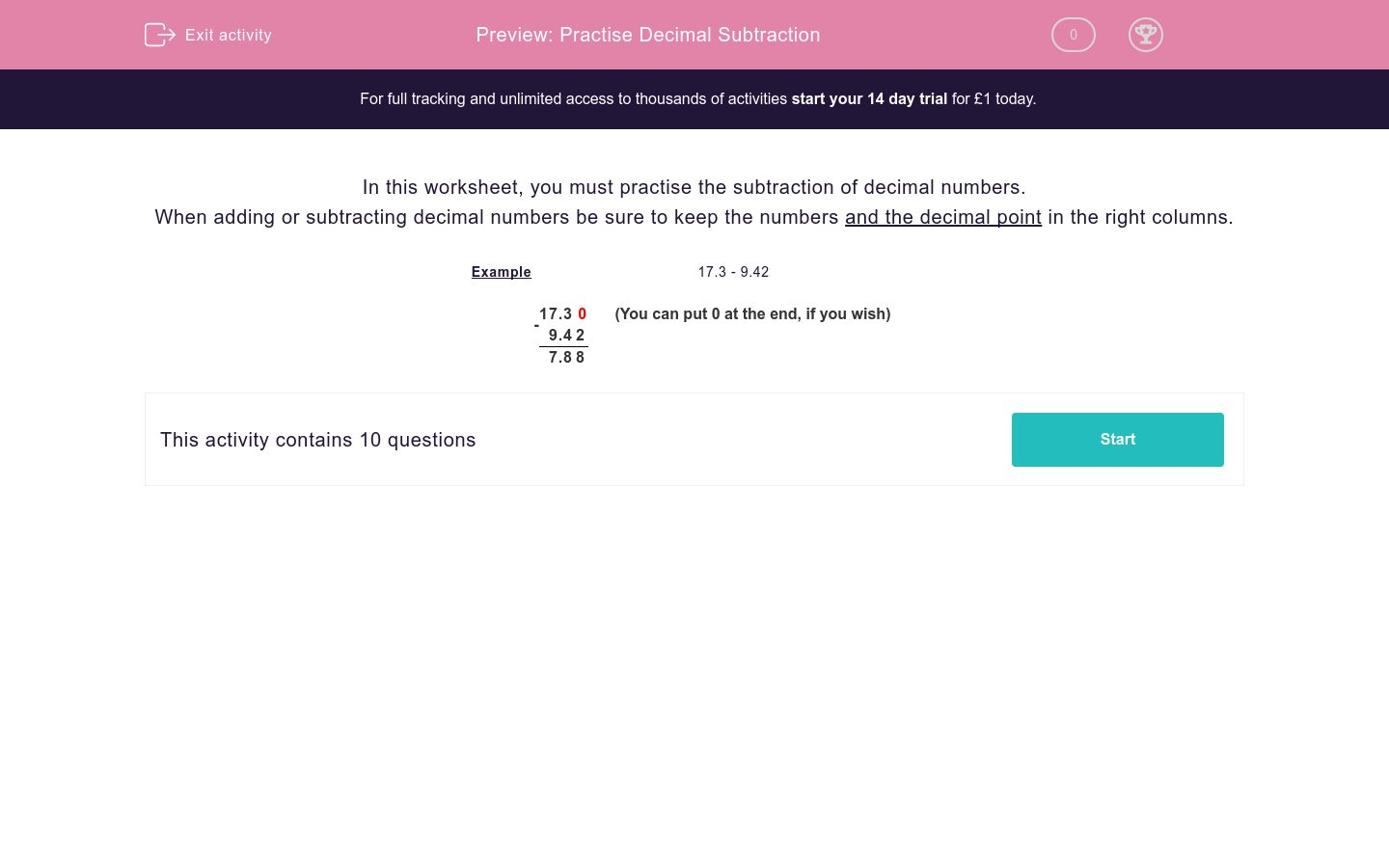# Practise Decimal Subtraction

In this worksheet, students practise subtraction of decimals.Key stage:  KS 2

Curriculum topic:   Maths and Numerical Reasoning

Curriculum subtopic:   Decimals

Difficulty level:### QUESTION 1 of 10

In this worksheet, you must practise the subtraction of decimal numbers.

When adding or subtracting decimal numbers be sure to keep the numbers and the decimal point in the right columns.

Example

17.3 - 9.42

 - 1 7 . 3 0 (You can put 0 at the end, if you wish) 9 . 4 2 7 . 8 8
---- OR ----